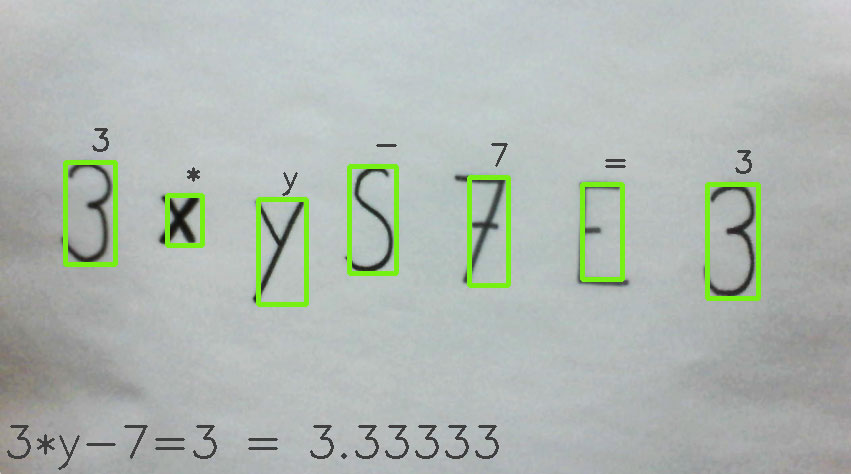# AI Equation Solver through OCRAs a proof of concept of AI for Interactive Media, an idea sprung to mind to build an optical equation solver. Using a camera and computer vision (OpenCV), we built an app which detected written letters and signs real-time. A multilayered perceptron neural network would then classify an image chunk as a character using optical character recognition (OCR), which could then be used in our equation solver. With this solution, a user can write close to any expression or equation containing known operators and let the application compute an answer.

In short, the process is divided into three parts:

1. Preprocessing - Each image in a read dataset contains a character which is converted into a string of bits by thresholding the image. The image is then cropped and rescaled before storing the result into a text file and which is used in the following step

2. Training - The processed dataset is used as the input for the training of the MLP-neural network. The result is exported and used in the application.

3. Application - The application uses a camera to register characters. The neural network guesses the classification of the characters in each frame. If the registered characters define a valid mathematical expression, the application calculates the result of the expression and dispose it to the user